library: libGeom#include "TGeoTube.h"

# TGeoCtub

class description - source file - inheritance tree (.pdf)

## class TGeoCtub : public TGeoTubeSeg

Inheritance Chart:
 TObject
<-
TNamed
<-
TGeoShape
<-
TGeoBBox
<-
TGeoTube
<-
TGeoTubeSeg
<-
TGeoCtub
```
public:
TGeoCtub()
TGeoCtub(Double_t rmin, Double_t rmax, Double_t dz, Double_t phi1, Double_t phi2, Double_t lx, Double_t ly, Double_t lz, Double_t tx, Double_t ty, Double_t tz)
TGeoCtub(const char* name, Double_t rmin, Double_t rmax, Double_t dz, Double_t phi1, Double_t phi2, Double_t lx, Double_t ly, Double_t lz, Double_t tx, Double_t ty, Double_t tz)
TGeoCtub(Double_t* params)
TGeoCtub(const TGeoCtub&)
virtual ~TGeoCtub()
static TClass* Class()
virtual void ComputeBBox()
virtual void ComputeNormal(Double_t* point, Double_t* dir, Double_t* norm)
virtual Bool_t Contains(Double_t* point) const
virtual Double_t DistFromInside(Double_t* point, Double_t* dir, Int_t iact = 1, Double_t step = TGeoShape::Big(), Double_t* safe = 0) const
virtual Double_t DistFromOutside(Double_t* point, Double_t* dir, Int_t iact = 1, Double_t step = TGeoShape::Big(), Double_t* safe = 0) const
virtual TGeoVolume* Divide(TGeoVolume* voldiv, const char* divname, Int_t iaxis, Int_t ndiv, Double_t start, Double_t step)
virtual Double_t GetAxisRange(Int_t iaxis, Double_t& xlo, Double_t& xhi) const
virtual Int_t GetByteCount() const
virtual TGeoShape* GetMakeRuntimeShape(TGeoShape* mother, TGeoMatrix* mat) const
const Double_t* GetNhigh() const
const Double_t* GetNlow() const
virtual Int_t GetNmeshVertices() const
Double_t GetZcoord(Double_t xc, Double_t yc, Double_t zc) const
virtual void InspectShape() const
virtual TClass* IsA() const
TGeoCtub& operator=(const TGeoCtub&)
virtual Double_t Safety(Double_t* point, Bool_t in = kTRUE) const
void SetCtubDimensions(Double_t rmin, Double_t rmax, Double_t dz, Double_t phi1, Double_t phi2, Double_t lx, Double_t ly, Double_t lz, Double_t tx, Double_t ty, Double_t tz)
virtual void SetDimensions(Double_t* param)
virtual void SetPoints(Double_t* buff) const
virtual void SetPoints(Float_t* buff) const
virtual void ShowMembers(TMemberInspector& insp, char* parent)
virtual void Streamer(TBuffer& b)
void StreamerNVirtual(TBuffer& b)
```

### Data Members

```
protected:
Double_t fNlow   normal to lower cut plane
Double_t fNhigh  normal to highet cut plane
```

## Class Description

``` TGeoTube - cylindrical tube class. It takes 3 parameters :

_____________________________________________________________________________
```
/*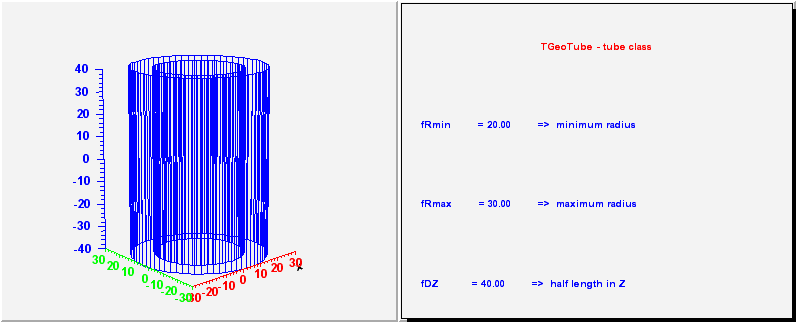*/

/*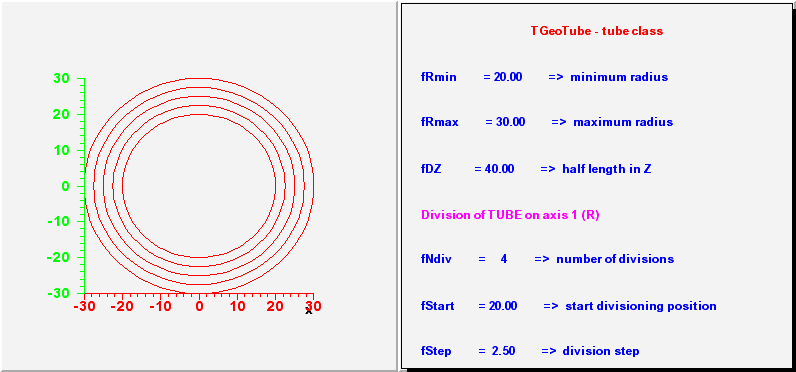*/

/*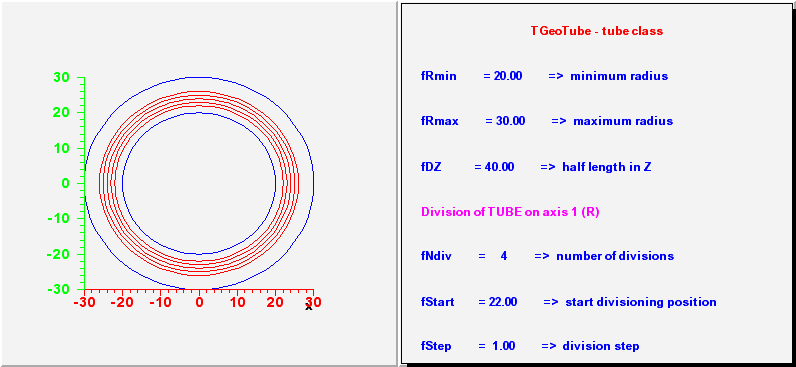*/

/*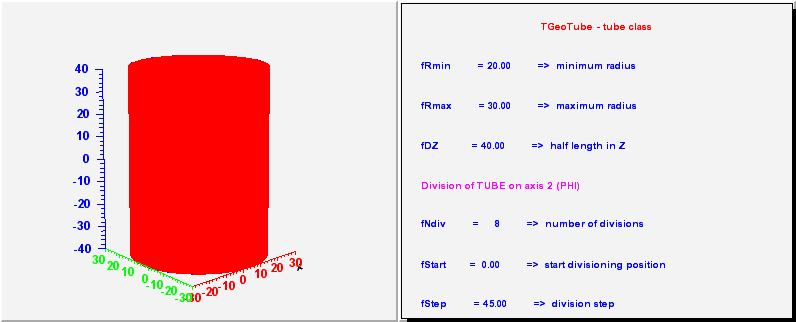*/

/*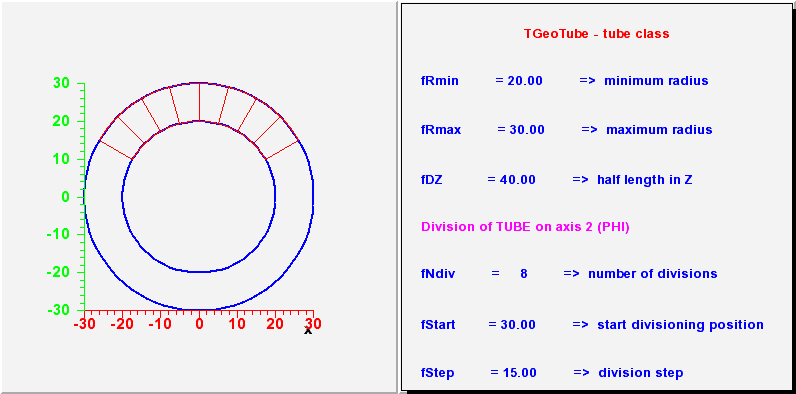*/

/*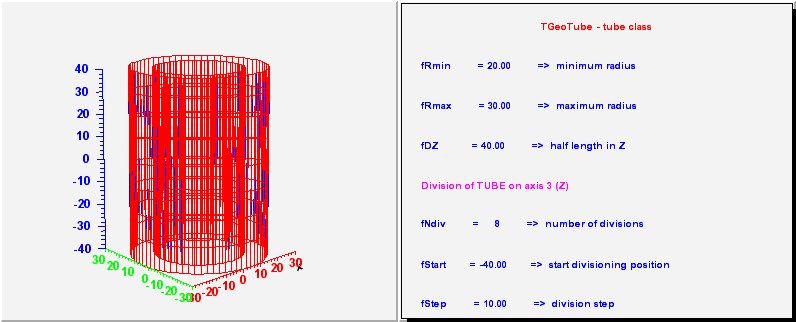*/

/*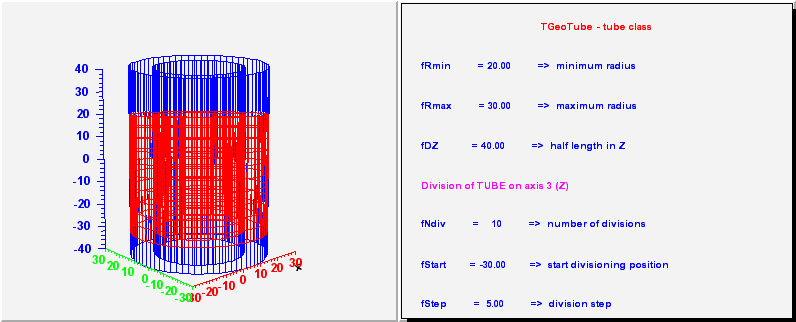*/

```_____________________________________________________________________________
TGeoTubeSeg - a phi segment of a tube. Has 5 parameters :
- the same 3 as a tube;
- first phi limit (in degrees)
- second phi limit

_____________________________________________________________________________
```
/**/

/**/

/**/

/**/

```_____________________________________________________________________________
TGeoCtub - a tube segment cut with 2 planes. Has 11 parameters :
- the same 5 as a tube segment;
- x, y, z components of the normal to the -dZ cut plane in
point (0, 0, -dZ);
- x, y, z components of the normal to the +dZ cut plane in
point (0, 0, dZ);

_____________________________________________________________________________
```
/**/

TGeoCtub()
``` default ctor
```

TGeoCtub(Double_t rmin, Double_t rmax, Double_t dz, Double_t phi1, Double_t phi2, Double_t lx, Double_t ly, Double_t lz, Double_t tx, Double_t ty, Double_t tz) :TGeoTubeSeg(rmin, rmax, dz, phi1, phi2)
``` ctor
```

TGeoCtub(const char *name, Double_t rmin, Double_t rmax, Double_t dz, Double_t phi1, Double_t phi2, Double_t lx, Double_t ly, Double_t lz, Double_t tx, Double_t ty, Double_t tz) :TGeoTubeSeg(name, rmin, rmax, dz, phi1, phi2)
``` ctor
```

TGeoCtub(Double_t *params) :TGeoTubeSeg(0,0,0,0,0)
``` ctor with parameters
```

~TGeoCtub()
``` dtor
```

void ComputeBBox()
``` compute minimum bounding box of the ctub
```

void ComputeNormal(Double_t *point, Double_t *dir, Double_t *norm)
``` Compute normal to closest surface from POINT.
```

Bool_t Contains(Double_t *point) const
``` check if point is contained in the cut tube
check the lower cut plane
```

Double_t GetAxisRange(Int_t iaxis, Double_t &xlo, Double_t &xhi) const
``` Get range of shape for a given axis.
```

Double_t GetZcoord(Double_t xc, Double_t yc, Double_t zc) const
``` compute real Z coordinate of a point belonging to either lower or
higher caps (z should be either +fDz or -fDz)
```

Double_t DistFromOutside(Double_t *point, Double_t *dir, Int_t iact, Double_t step, Double_t *safe) const
``` compute distance from outside point to surface of the cut tube
```

Double_t DistFromInside(Double_t *point, Double_t *dir, Int_t iact, Double_t step, Double_t *safe) const
``` compute distance from inside point to surface of the cut tube
```

TGeoVolume* Divide(TGeoVolume * /*voldiv*/, const char * /*divname*/, Int_t /*iaxis*/, Int_t /*ndiv*/, Double_t /*start*/, Double_t /*step*/)

TGeoShape* GetMakeRuntimeShape(TGeoShape *mother, TGeoMatrix * /*mat*/) const
``` in case shape has some negative parameters, these has to be computed
in order to fit the mother
```

void InspectShape() const
``` print shape parameters
```

Double_t Safety(Double_t *point, Bool_t in) const
``` computes the closest distance from given point to this shape, according
to option. The matching point on the shape is stored in spoint.
```

void SetCtubDimensions(Double_t rmin, Double_t rmax, Double_t dz, Double_t phi1, Double_t phi2, Double_t lx, Double_t ly, Double_t lz, Double_t tx, Double_t ty, Double_t tz)
``` set dimensions of a cut tube
```

void SetDimensions(Double_t *param)

void SetPoints(Double_t *buff) const
``` create sphere mesh points
```

void SetPoints(Float_t *buff) const
``` create sphere mesh points
```

Int_t GetNmeshVertices() const
``` Return number of vertices of the mesh representation
```

### Inline Functions

```                  Int_t GetByteCount() const
const Double_t* GetNlow() const
const Double_t* GetNhigh() const
TClass* Class()
TClass* IsA() const
void ShowMembers(TMemberInspector& insp, char* parent)
void Streamer(TBuffer& b)
void StreamerNVirtual(TBuffer& b)
TGeoCtub TGeoCtub(const TGeoCtub&)
TGeoCtub& operator=(const TGeoCtub&)
```

Author: Andrei Gheata 24/10/01
Last update: root/geom:\$Name: \$:\$Id: TGeoTube.cxx,v 1.50 2004/12/07 14:24:57 brun Exp \$